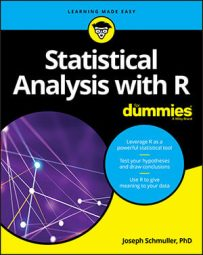##### Statistical Analysis with R For DummiesThe grammar-of-graphics approach takes considerably more effort when plotting the values of a t-distribution than base R. But follow along and you'll learn a lot about ggplot2.

You start by putting the relevant numbers into a data frame:

```t.frame = data.frame(t.values, ``` `df3 = dt(t.values,3),`

```df10 = dt(t.values,10), ``` ```std_normal = dnorm(t.values)) ``` The first six rows of the data frame look like this:

```> head(t.frame) ``` ```t.values df3 df10 std_normal ``` ```1 -4.0 0.009163361 0.002031034 0.0001338302 ``` ```2 -3.9 0.009975671 0.002406689 0.0001986555 ``` `3 -3.8 0.010875996 0.002854394 0.0002919469`

```4 -3.7 0.011875430f 0.003388151 0.0004247803 ``` ```5 -3.6 0.012986623 0.004024623 0.0006119019 ``` `6 -3.5 0.014224019 0.004783607 0.0008726827`

That's a pretty good-looking data frame, but it's in wide format. `ggplot() `prefers long format — which is the three columns of density-numbers stacked into a single column. To get to that format — it's called reshaping the data — make sure you have the `reshape2 `package installed. Select its check box on the Packages tab and you're ready to go.

Reshaping from wide format to long format is called melting the data, so the function is

```t.frame.melt <- melt(t.frame,id="t.values") ``` The `id `argument specifies that `t.values `is the variable whose numbers don't get stacked with the rest. Think of it as the variable that stores the data. The first six rows of `t.frame.melt `are:

```> head(t.frame.melt) ``` ```t.values variable value ``` ```1 -4.0 df3 0.009163361 ``` `2 -3.9 df3 0.009975671`

```3 -3.8 df3 0.010875996 ``` ```4 -3.7 df3 0.011875430 ``` ```5 -3.6 df3 0.012986623 ``` ```6 -3.5 df3 0.014224019 ``` It's always a good idea to have meaningful column names, so . . .

`> colnames(t.frame.melt)= c("t","df","density")`

```> head(t.frame.melt) ``` ```t df density ``` ```1 -4.0 df3 0.009163361 ``` `2 -3.9 df3 0.009975671`

```3 -3.8 df3 0.010875996 ``` `4 -3.7 df3 0.011875430`

```5 -3.6 df3 0.012986623 ``` `6 -3.5 df3 0.014224019`

Now for one more thing before you start on the graph. This is a vector that will be useful when you lay out the x-axis:

```x.axis.values <- seq(-4,4,2) ``` Begin with ggplot():

```ggplot(t.frame.melt, aes(x=t,y=f(t),group =df)) ``` The first argument is the data frame. The aesthetic mappings tell you that `t` is on the x-axis, density is on the y-axis, and the data falls into groups specified by the df variable.

This is a line plot, so the appropriate `geom `function to add is `geom_line`:

`geom_line(aes(linetype=df))`

`Geom` functions can work with aesthetic mappings. The aesthetic mapping here maps `df` to the type of line.

Rescale the x-axis so that it goes from –4 to 4, by twos. Here's where to use that `x.axis.`values vector:

```scale_x_continuous(breaks=x.axis.values,labels=x.axis.values) ``` The first argument sets the breakpoints for the x-axis, and the second provides the labels for those points. Putting these three statements together

```ggplot(t.frame.melt, aes(x=t,y=density,group =df)) + ``` ```geom_line(aes(linetype=df)) + ``` ```scale_x_continuous(breaks = x.axis.values,labels = x.axis.values) ``` results in the following figure. One of the benefits of ggplot2 is that the code automatically produces a legend.

You still have some work to do. First of all, the default `linetype `assignments are not what you want, so you have to redo them:

```scale_linetype_manual(values = c("dotted","dashed","solid"), ``` `labels = c("3","10", expression(infinity)))`

The four statements

```ggplot(t.frame.melt, aes(x=t,y=density,group =df)) + ``` ```geom_line(aes(linetype=df)) + ``` ```scale_x_continuous(breaks = x.axis.values,labels = x.axis.values)+ ``` ```scale_linetype_manual(values = c("dotted","dashed","solid"), ``` `labels = c("3","10", expression(infinity)))`

produce the following.

As you can see, the items in the legend are not in the order that the curves appear at their centers. A graph is more comprehensible when the graph elements and the legend elements are in sync. ggplot2 provides `guide `functions that enable you to control the legend's details. To reverse the order of the linetypes in the legend, here's what you do:

```guides(linetype=guide_legend(reverse = TRUE)) ``` Putting all the code together, finally, yields the following figure.

```ggplot(t.frame.melt, aes(x=t,y=density,group =df)) + ``` ```geom_line(aes(linetype=df)) + ``` ```scale_x_continuous(breaks = x.axis.values,labels = x.axis.values)+ ``` ```scale_linetype_manual(values = c("dotted","dashed","solid"), ``` ```labels = c("3","10", expression(infinity)))+ ``` `guides(linetype=guide_legend(reverse = TRUE))`

Base R graphics versus ggplot2: It's like driving a car with a standard transmission versus driving with an automatic transmission!